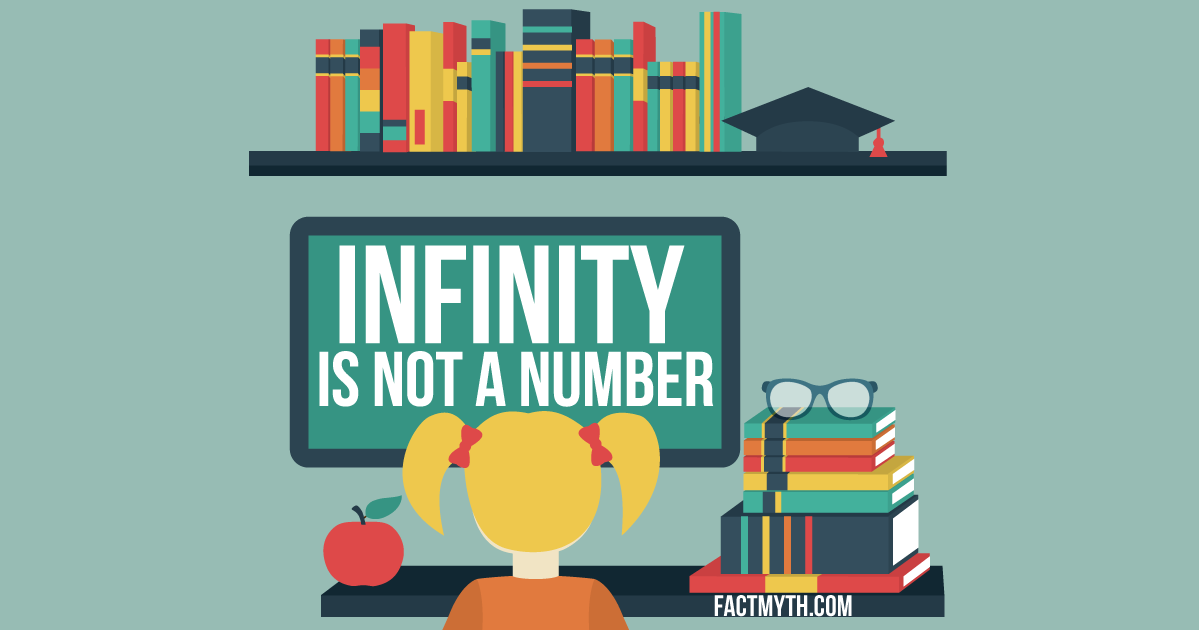# Infinity is a Number myth## Is Infinity a Number?

Infinity (∞) isn’t a number; it’s a concept. It describes something that can’t be expressed by a “finite” natural or real number. Finite systems are “bound”. Infinite systems are “not bound” and have more elements than something finite. Despite this, in mathematics, infinity is sometimes treated as if it were a “really big” number for practical purposes. Luckily, infinity doesn’t need to be a number to be useful in everyday physics and mathematics. There are many different types of infinity, and many ways to work with infinity and infinitesimals.

## What are Irrational Numbers?

If mathematics results in an infinite string of numbers, the string is said to be an “irrational number”. The square roots of prime numbers are infinite irrational numbers (and the general weirdness of primes makes them a good fit for cryptography).

In real life, irrational numbers symbolized by π (Pi) and √2 (square root of two) are very useful for calculating perfect shapes (for instance a perfect curve, like the one found in a circle, is only calculable with an irrational “infinite” number). Infinity is a concept that can be estimated mathematically by numbers, or represented by symbols and functions. We can’t write out the square root of two, so instead we just use √2, we can’t write the irrational string of numbers that is Pi, so we just use π, we can’t write out an infinite set, but we can define {…, -1, 0, 1, 2, …} and put it to use.Infinity is a concept, not a number. Image Source

## Is Infinity Real?

Despite the fact that we can’t express it fully, infinity is real and exists both in mathematics and in real life. In both we can apply things like calculus, set theory, and limits to help us understand that infinity is a workable concept and not a sticking point. As noted above, the best proof is the everyday application, where we use the concept of infinity for everything from Pi, to square roots, to mass-energy equivalence.

Analysis, computing, physics, and more all work with limits and infinities on a regular basis with success.

Watch the video below for an introduction on infinity, and if you want to have your mind blown to infinity and beyond, check out our page on “the different types of infinity“.

How big is infinity? – Dennis Wildfogel.

Conclusion

We may think of infinity in terms of “really big numbers” in math, but infinity is a concept, not an actual number.

References